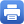Columbia Home

### MATH GR8210 – PARTIAL DIFFERENTIAL EQUATIONS

Day/Time: TR 11:40am-12:55pm
Room: 407, Mathematics Hall

Title: Ancient Solutions to Geometric Flows

Abstract: Some of the most important problems in evolution partial differential equations are related to the understanding of singularities. This usually happens through a blow-up procedure near the potential singularity which uses the scaling properties of the partial differential equation involved. In the case of a parabolic equation the blow-up analysis often leads to special solutions which are defined for all time −∞ < t ≤ T for some T ≤ +∞. We refer to them as ancient solutions. The classification of such solutions often sheds new insight to the singularity analysis. In some flows it is also important for performing surgery near a singularity.

In this course we will discuss uniqueness theorems for ancient solutions to nonlinear partial differential equations and in particular to geometric flows such as Mean curvature flow and Ricci flow. This subject has recently seen major advancements. Emphasis will be given to the techniques which have been developed to study these subjects, as they have a wider scope of applicability beyond the special parabolic equations discussed in this course.

The following is a brief outline of the course which may change depending on the students demands and as the course progresses:
(1) Introduction.
(2) Ancient solutions to the semi-linear heat equation.
(3) Liouville theorems for the Navier-Stokes equations.
(4) The classification of ancient compact solutions to curve shortening flow.
(5) Ancient compact non-collapsed solutions to Mean curvature flow.
(6) The classification of ancient compact solutions to the Ricci flow on S^2.
(7) The classification of ancient compact solutions to the Ricci flow on S^3.

Background: Graduate courses on: (i) elliptic and parabolic PDE and (ii) Differential geometry.Print this page